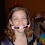## Friday, July 18, 2008

### What Do You Know About the Olympics

What is the maximal height a pole vaulter could theoretically jump? Would it ever be possible that a pole vaulter passes the height of 10 meter?

1.Yes, he could jump higher than 10 meters. There is no "theoretical" maximum height. The pole acts as a spring, storing energy put in by the jumper at the beginning, as he runs and plants the pole. The maximum height would depend on the running speed of the jumper and the physical characteristics of the pole.

I'm not sure if that is the answer you had in mind, Mike.

2.Yes, you've got the right idea behind you, but I would argue that there are physical limitations as to what humans can do, even if you make the perfect pole. The only caveat here is I assume you're playing on earth.

At the point that the pole vaulter is going to jump, the total energy E = 1/2 * M * v^2 + M * g * l / 2. Here M is the mass of the pole vaulter (we neglect the mass of the pole with respect to the mass of the pole vaulter), v the speed of the pole vaulter, g is gravity (9.8 m/s^2) and l the length of the pole vaulter. We assumed that the center of mass of the pole vaulter is halfway his length.

If the pole vaulter is at the highest point, his kinetic energy is about zero, since he almost doesn't move anymore at that point. The total energy E is than equal to the potential energy, E = M * g * h, where h is the height of the jump.

So 1/2 * M * v^2 + 1/2 * M * g * l = M * g * h. From this it follows that h = (l + v^2 / g) / 2.

So a high running speed is of great importance for getting high jump. The maximal reachable running speed can be estimated by realizing that the world's best athletes can run 100 meters in 10 seconds. The pole vaulter will probably not go faster.

So for a jump as high as possible we take v = 10 m/s. Suppose the pole vaulter has a length of 2 meter. Then h = (2 + 10*10 / 9.81) / 2 = 6.1 meter. By pushing yourself from the pole it is possible to put some additional energy into the system. As a result it could be possible to reach somewhat higher than 6.1 meter.

So, to see someone hit 10 meters (on earth) would be just about impossible.

3.How about somebody from the Paralypics. Somebody with artificial legs made out of that carbon-fiber would have significant running ability if he trained properly, probably could even pick up more speed then 100meters/10sec. That and the lighter legs would allow the athlete to possibly get better height. He might hit 10meters.

4.I suppose that's possible, but that means they need to hit 13.2 m/s. If you were capable of hitting 13.2 m/s, you could the 100 meter dash in 7.5 seconds.

That seems to be out of reach for unassisted runners.

According to wikipedia, the world record for pole vaulting is 6.14 meters.

http://en.wikipedia.org/wiki/World_Record_progression_Pole_Vault_men

Leave your answer or, if you want to post a question of your own, send me an e-mail. Look in the about section to find my e-mail address. If it's new, I'll post it soon.

Please don't leave spam or 'Awesome blog, come visit mine' messages. I'll delete them soon after.

Enter your Email and join hundreds of others who get their Question of the Day sent right to their mailbox

The Lamplight Manor Puzz 3-D